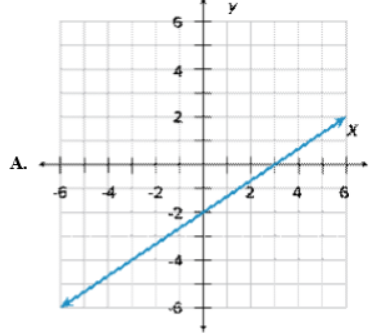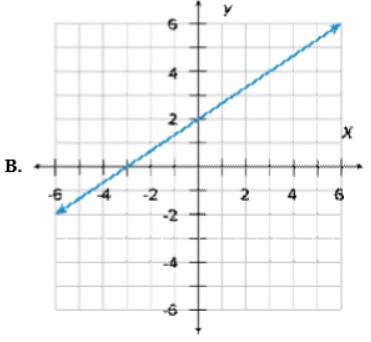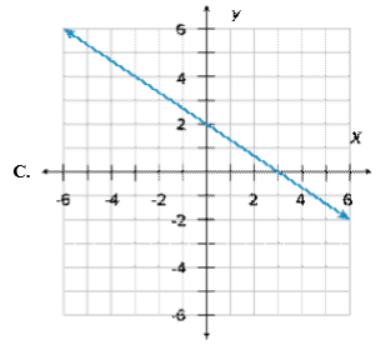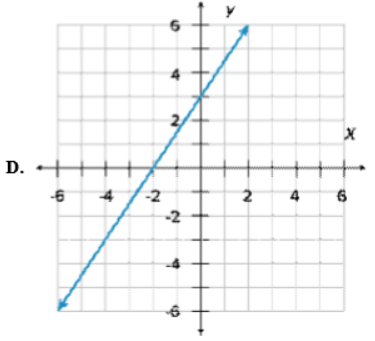# Which of the following is the graph of 2x + 3y = 6?

Which of the following is the graph of 2x + 3y = 6?